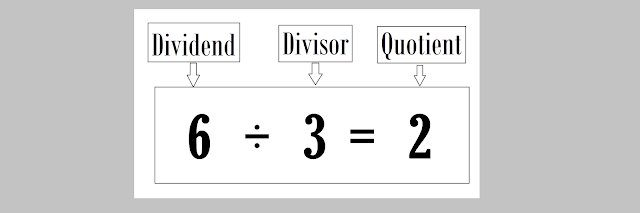### What is Divisor? Definition with Examples-

A Divisor is a number that divides another number completely or with a Reminder.
for example-Fig.1- Example of Dividend, Divisor, and Quotient

In Fig.1, On Dividing 6 by 3, we get 2. Here 3 is the number which divides 6 completely into 2 Parts and is known as the Divisor.
Note:- Its sound like Divisor and Factor are both same but it's not actually. A divisor is a number that divides another number completely or with a reminder but,
The factor is the number that divides another number completely with no Reminder.
For eg.-Fig.2- Equation with Reminder
Here,
by dividing 21 by 4, we get 5 and Reminder 1. So, here 4 is a Divisor but not a factor.
But, in Fig.1, we divide 6 by 3 and Got 2 with no reminder. so, 3 is a Divisor and a factor of 6.

Note 2- A factor is always a Divisor but A divisor can't be always a factor.

### Dividend

A dividend is an amount that you want to Divide. dividend ÷ divisor = quotient. For eg. 25 ÷ 5 = 5, Here 25 is  Dividend.

### Quotient

It is a result obtained by dividing a number by another.